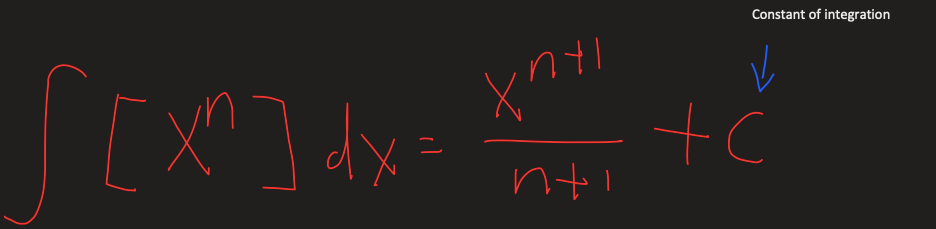top of page

# How to solve the definite and indefinite integral of a quadratic function

Updated: May 8, 2021

It is basically function which has a structure of: f(x) = ax^2+bx+c where (a) is not equal to zero.

In a quadratic function, the greatest power of the variable is 2. The graph of a quadratic function is a parabola.

example:Finding the integral of a function from x1 to x2 could be understood as finding the area between the x axis the curve of the function from x1 to x2. Although there are methods of finding the area by hand, I'll teach you a basic method which will work as long as there aren't any fractions of square roots in the function.

Here is a method of solving an indefinite integral where x1 and x2 are not defined, in indefinite integrals, you always have to add a constant at the end(it could be any number).Here is an example:If there is a constant in the function, think of it as the constant times X to the power of zero, since anything to the power of 0 is 1 (except for zero).

Definite integration:

From before we can know that x to the power of n = x to the power of n+1 divided by n+1But now we evaluate the answer from the two numbers (the x1 and x2 of the area we are looking for). Here is an example:Lets take a look at a harder example:However, note that this method doesn't work for all functions for there might be a invalid number. Like f(5/x) where x = 0 is an invalid number(you can't divide by zero).

See All
bottom of page